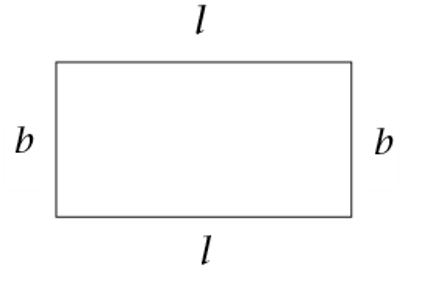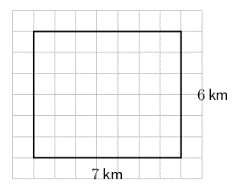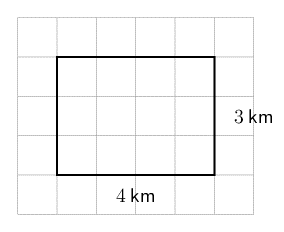Home Practice
For learners and parents For teachers and schools
Textbooks
Full catalogue
Pricing SupportLog in

We think you are located in United States. Is this correct?

# Area and perimeter of rectangles

## 16.4 Area and perimeter of rectangles

The opposite sides of a rectangle are equal in length. The perimeter of a rectangle is equal to the sum of the lengths of its four sides.\begin{align} \text{Perimeter of a rectangle } &= l + b + l + b \\ &= 2l + 2b \\ &= 2(l + b) \end{align}

The area of a rectangle is equal to the product of its length and breadth.

$\text{Area of a rectangle } = l \times b$

## Worked example 16.8: Calculating the perimeter of a rectangle### Calculate the perimeter of the rectangle.

Find the sum of all four sides of the rectangle.

$\text{Perimeter of the rectangle } = 7 + 6 + 7 + 6 = 2 (7 + 6) = 2(13) = 26 \text{ km}$

### Convert the units to give the answer in metres.

$$1 \text{ km} = 1\ 000 \text{ m}$$, so we get $$26 \text{ km} = 26 \times 1\ 000 = 26\ 000 \text{ m}$$.

$$\text{Perimeter of the rectangle } = 26\ 000 \text{ m}$$.

## Worked example 16.9: Calculating the area of a rectangle

Calculate the area of the rectangle.### Calculate the area of the rectangle.

To calculate the area if the rectangle, we use the formula:

\begin{align} \text{Area of the rectangle } &= l \times b \\ &= 4 \times 3 \\ &= 12 \end{align}

$$\text{Area of the rectangle} = 12 \text{ km}^2$$.